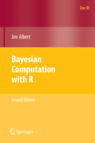#Bayesian Computation With R

##### by Jim Albert

1. An Introduction to R
2. Introduction to Bayesian Thinking
3. Single-Parameter Models
4. Multiparameter Models
5. Introduction to Bayesian Computation
6. Markov Chain Monte Carlo Methods
7. Hierarchical Modeling
8. Model Comparision
9. Regression Models
10. Gibbs Sampling
11. Using R to Interface with WinBUGS